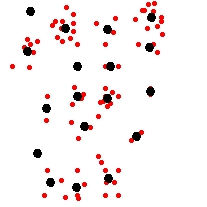Open Table of Contents

Thin (Generalize) Points

Go to ToolBox Implementation

Reduces the number of points in a point dataset based on their spatial location.

• Points that are closer to each other than the tolerance specified will be converted to a single point.
• The values in the field specified will be summarized for each cluster and saved in the point representing this cluster.
• Optionally a link from the original points to the generalized one can be added.

Inputs:

• A Point feature class
• Generalization tolerance - should be smaller than 20% of the smaller side of the extent envelope of the input dataset.
• Data field - if specified the output feature class will have a field in which the value for each resulting point will be the sum of the values of the points pertaining to this cluster.

Outputs:

• New point feature class. Fields:
• [ET_Count] - the number of points from the input feature class represented by each output point
• Value Field - the sum of the values in the user specified field
• [ET_Link] - added only if the "Add Link from the original points" option is selected.

Notes:

• If no Generalization tolerance is specified, the tolerance will be set to 0 - only the exact duplicates will be removed

Examples:

 Input Dataset Result Dataset labeled with the number of points they represent (ET_Count field) OverlayCommand line syntax

ET_GPThinPoints<input_dataset> <out_feature class> <tolerance> {add_link} {value_field}

Parameters

Expression Explanation
<input_dataset> A  Point feature class or feature layer
<out_feature_ class> A String - the full name of the output feature class (A feature class with the same full name should not exist)
<tolerance> A Double representing the Generalization tolerance.
{add_link} A Boolean. If TRUE, a link field is added to both Original and Generalized points. The values in this field ("ET_Link") can be used to back link the generalized points to the original ones.
{value_field} A String representing  the Data field.

Scripting syntax

ET_GPThinPoints (input_dataset,  out_feature_ class, tolerance, add_link, value_field )

See the explanations above:
<> - required parameter
{} - optional parameter

 Copyright © Ianko Tchoukanski